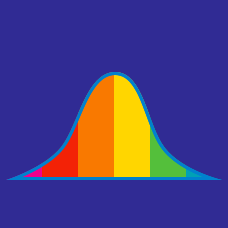Probability

# Data - Median

$\{ 3, 7, 10, 25, 40 \}$

What is the median of the above set?

What is the median income in the following data? \begin{aligned} &\text{Income} &\text{No. of}\\ &\text{in RS.} &\text{persons}\\ \\ &2000 & 9\\ &2100 & 36\\ &2200 & 12\\ &2300 & 22\\ &2400 & 16\\ &2500 & 6 \end{aligned}

9 students took a test that was scored out of 10.

2 of them got a score of 0.
3 of them got a score of 4.
4 of them got a score of 8.

What is the median score?

The weekly salaries of five employees of a company are $250,$220, $270,$150, and \$200, respectively. What is the median of their weekly salaries?

$\{ 4, 21, 8 \}$

What is the median of the above set?

×